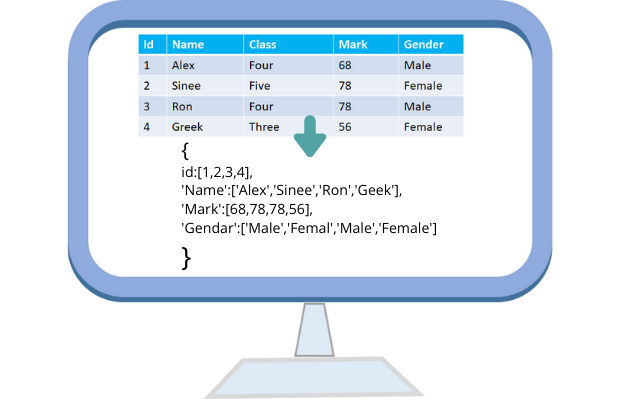# to_dict()

Pandas Pandas input output``DataFrame.to_dict(orient='dict', into=<class 'dict'>)``
`orient`
dict ( default ) {column -> {index -> value}}
list {column -> [values]}
series {column -> series[values]}
split {'index -> [index], 'columns' -> [columns], 'data' -> [values]}
records [{column -> value}, … , {column -> value}]
index {index -> {column -> value}}

`into`
class ( default dict ) Mapping subclass used for all Mappings in the return value
See all examples below.

## Creating DataFrame

``````df=pd.DataFrame(data={'id': [1, 2, 3],
'name': ['John Deo', 'Max Ruin', 'Arnold'],
'class': ['Four', 'Three', 'Three'],
'mark': [75, 85, 55],
'gender': ['female', 'male', 'male']})``````
Using this DataFrame we can create the Dictionary by using to_dict().
``````my_dict=df.to_dict()
print(my_dict)``````
Output is here
``````{'id': {0: 1, 1: 2, 2: 3},
'name': {0: 'John Deo', 1: 'Max Ruin', 2: 'Arnold'},
'class': {0: 'Four', 1: 'Three', 2: 'Three'},
'mark': {0: 75, 1: 85, 2: 55},
'gender': {0: 'female', 1: 'male', 2: 'male'}}``````

## orient='dict' ( default )

``my_dict=df.to_dict(orient='dict')``
``````{'id': {0: 1, 1: 2, 2: 3},
'name': {0: 'John Deo', 1: 'Max Ruin', 2: 'Arnold'},
'class': {0: 'Four', 1: 'Three', 2: 'Three'},
'mark': {0: 75, 1: 85, 2: 55},
'gender': {0: 'female', 1: 'male', 2: 'male'}}``````

## orient='list'

``my_dict=df.to_dict( orient='list')``
Output is here
``````{'id': [1, 2, 3],
'name': ['John Deo', 'Max Ruin', 'Arnold'],
'class': ['Four', 'Three', 'Three'],
'mark': [75, 85, 55],
'gender': ['female', 'male', 'male']}``````

## orient='series'

``my_dict=df.to_dict( orient='series')``
Output is here
``````{'id': 0    1
1    2
2    3
Name: id, dtype: int64, 'name': 0    John Deo
1    Max Ruin
2      Arnold
Name: name, dtype: object, 'class': 0     Four
1    Three
2    Three
Name: class, dtype: object, 'mark': 0    75
1    85
2    55
Name: mark, dtype: int64, 'gender': 0    female
1      male
2      male
Name: gender, dtype: object}``````

## orient='split'

``my_dict=df.to_dict( orient='split')``
Output is here
``````{'index': [0, 1, 2],
'columns': ['id', 'name', 'class', 'mark', 'gender'],
'data': [[1, 'John Deo', 'Four', 75, 'female'],
[2, 'Max Ruin', 'Three', 85, 'male'],
[3, 'Arnold', 'Three', 55, 'male']]}``````

## orient='records'

``my_dict=df.to_dict( orient='records')``
Output is here
``````[{'id': 1,
'name': 'John Deo',
'class': 'Four',
'mark': 75,
'gender': 'female'},
{'id': 2, 'name': 'Max Ruin', 'class': 'Three', 'mark': 85, 'gender': 'male'},
{'id': 3, 'name': 'Arnold', 'class': 'Three', 'mark': 55, 'gender': 'male'}]``````

## orient='index'

``my_dict=df.to_dict( orient='index')``
Output is here
``````{0: {'id': 1,
'name': 'John Deo',
'class': 'Four',
'mark': 75,
'gender': 'female'},
1: {'id': 2,
'name': 'Max Ruin',
'class': 'Three',
'mark': 85,
'gender': 'male'},
2: {'id': 3,
'name': 'Arnold',
'class': 'Three',
'mark': 55,
'gender': 'male'}}``````

## DataFrame from MySQL database

We will collect records from our sample student table in MySQL database and create the DataFrame. From the DataFrame by using to_dict() we will generate the dictionary.
Collect SQL dump of sample student table below.
Read more on MySQL with SQLAlchemy connection
``````import pandas as pd
from sqlalchemy import create_engine
my_conn = create_engine("mysql+mysqldb://userid:pw@localhost/my_db")
sql="SELECT * FROM student LIMIT 0, 3"
my_dict=df.to_dict()
my_dict``````
Output is here
``````{'id': {0: 1, 1: 2, 2: 3},
'name': {0: 'John Deo', 1: 'Max Ruin', 2: 'Arnold'},
'class': {0: 'Four', 1: 'Three', 2: 'Three'},
'mark': {0: 75, 1: 85, 2: 55},
'gender': {0: 'female', 1: 'male', 2: 'male'}}``````

## From Excel file to Dictionary

In above code we have created one DataFrame by taking data from a MySQL database table. We can create DataFrame by using any excel data or by using any csv (Comma Separated Value) file or from any other sources. ( check here to create a DataFrame from 8 different sources )
Once a DataFrame is created, then using that we can create dictionary output by using to_dict(). Here is one example to read one Excel file to a DataFrame and generate the string, you can explore other sources to create a DataFrame and finally generate dictionary.
``````df=pd.read_excel("D:\\my_data\\student.xlsx") # Path of the file.
df.to_dict()``````
``````df=pd.read_csv("D:\\my_data\\student.csv") # change the path
df.to_dict()``````

Subscribe to our YouTube Channel here

## Subscribe

* indicates required
Subscribe to plus2netplus2net.com